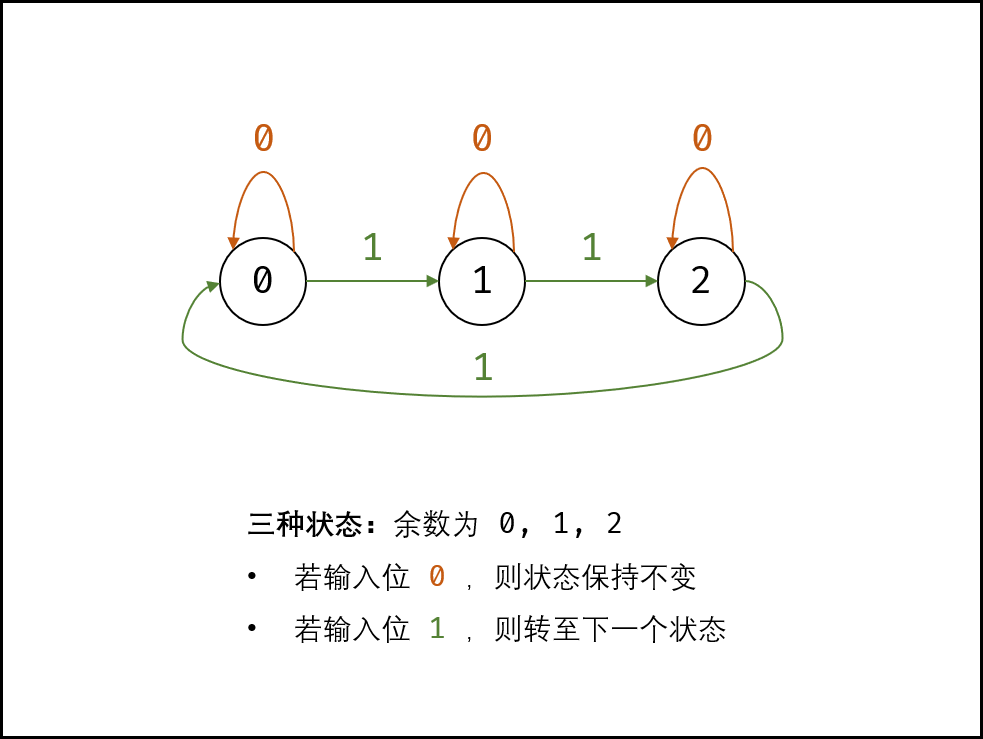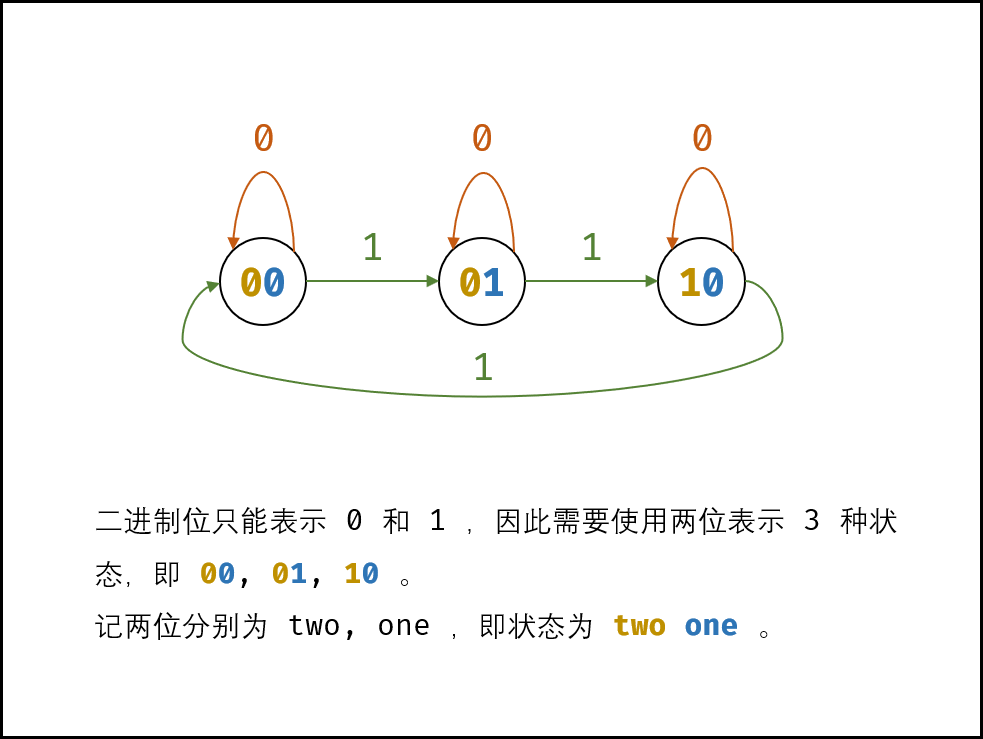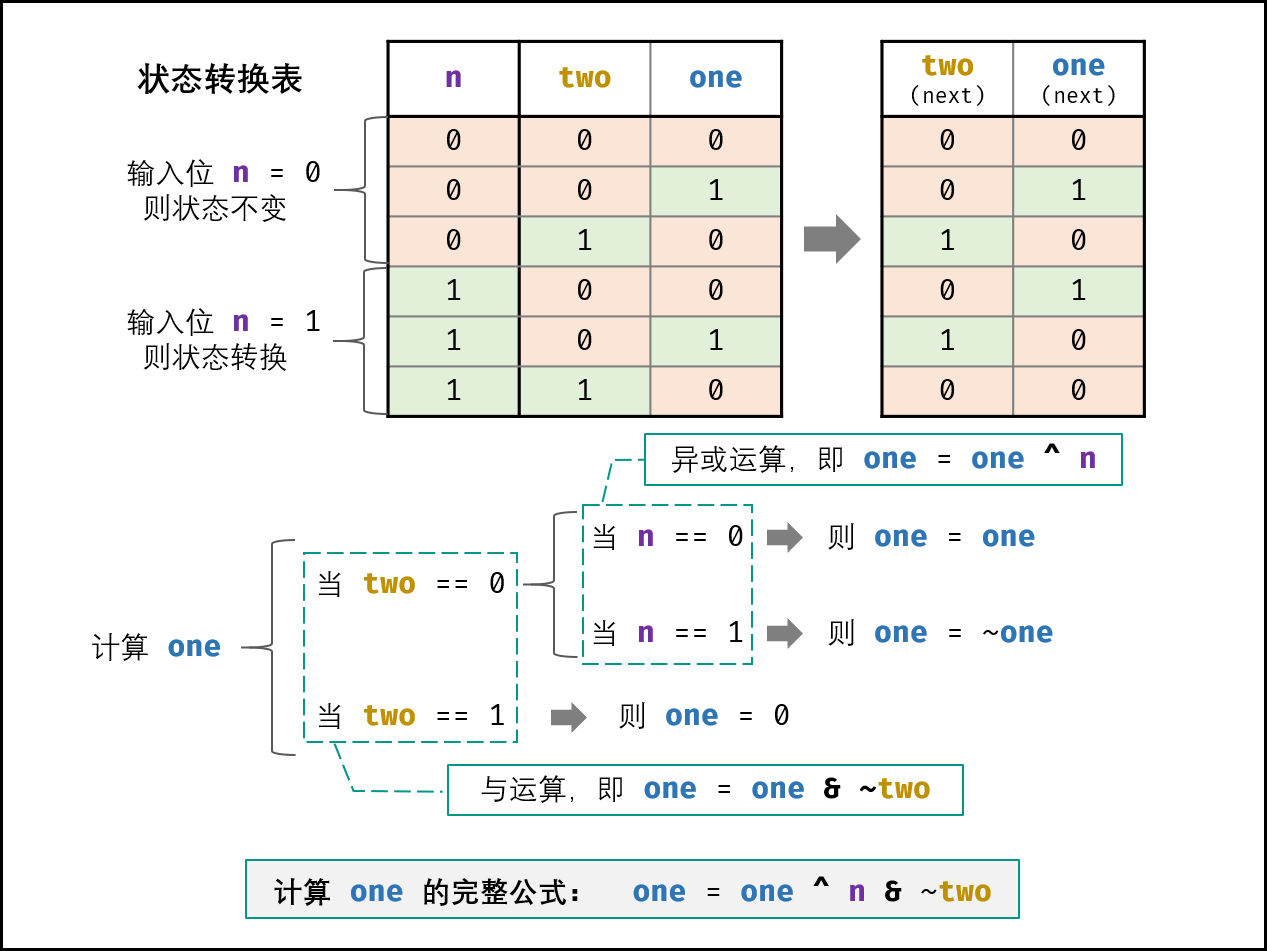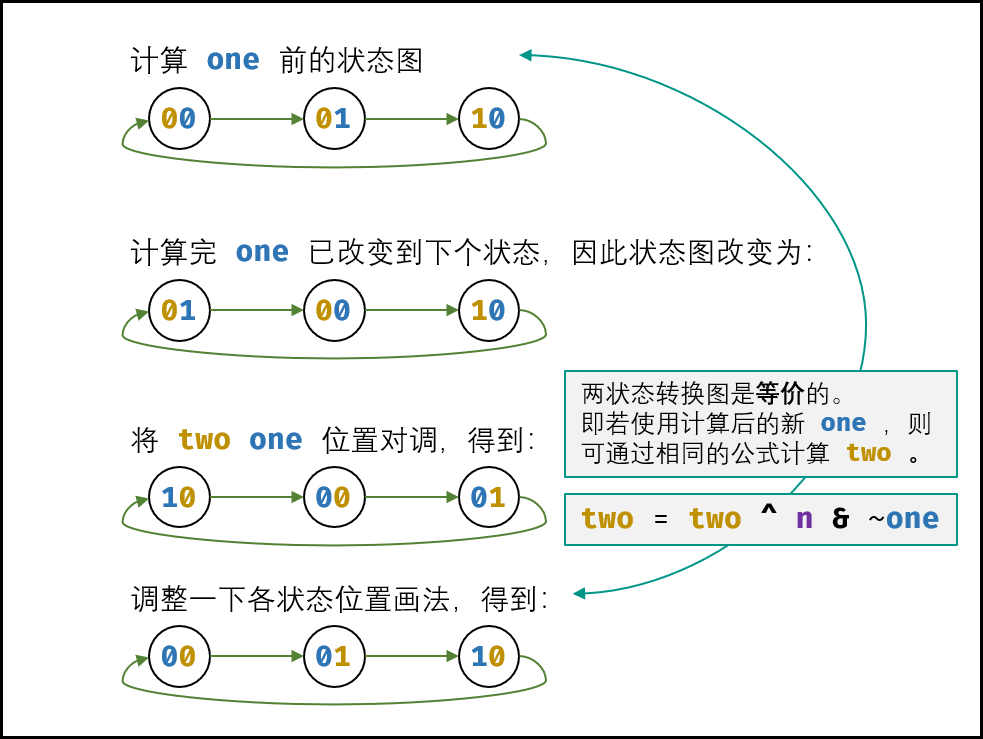# zrlong 的个人博客

X

## 解析

#### 有限状态自动机

• 输入二进制位1，则状态按如下顺序转换

• 输入二进制位0，则状态不变

0120• 异或运算：`x ^ 0 = x``x ^ 1 = ~x`
• 与运算：`x & 0 = 0``x & 1 = x`
• `~0 = -1``~1 = -2``1 & -1 = 1``1 & -2 = 0`
##### 计算one的方法：
``````if two == 0:
if n == 0:
one = one
if n == 1:
one = ~one
if two == 1:
one = 0
``````

``````if two == 0:
one = one ^ n
if two == 1:
one = 0
``````

``````one = one ^ n & ~two
``````##### 计算two的方法：``````two = two ^ n & ~one
``````

## 代码：

``````class Solution {
public int singleNumber(int[] nums) {
int ones = 0, twos = 0;
for(int num : nums){
ones = ones ^ num & ~twos;
twos = twos ^ num & ~ones;
}
return ones;
}
}
``````
##### 二进制各位相加，取余
``````class Solution {
public int singleNumber(int[] nums) {
int res = 0;;
int[] counts = new int;
for(int i = 0; i < nums.length; i++) {
for(int j = 0; j < 32; j++) {
counts[j] += nums[i] & 1; // 更新第 j 位
nums[i] >>>= 1; // 第 j 位 --> 第 j + 1 位
}
}
for(int i = 0; i < 32; i++) {
counts[i] %= 3; // 得到 只出现一次的数字 的第 (31 - i) 位
}
for(int i = 0; i < counts.length; i++) {
res <<= 1; // 左移 1 位
res |= counts[31 - i]; // 恢复第 i 位的值到 res
}
return res;
}
}
``````